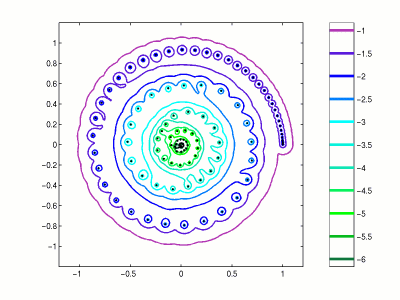Home | Introduction | Software | Examples | History | People | Bibliography | Applications | Theorems

## Pseudospectra of a Discretization of a Laser Integral EquationThis figure illustrates approximate pseudospectra of an integral equation from laser theory investigated by Landau [Lan77] in one of the earliest applications of pseudospectra. This example discretizes the infinite dimensional problem into a matrix of dimension N=600, and then projects this discretization onto an interesting invariant subspace of dimension 109.

Use the following MATLAB code compute a similar image using EigTool. To mimic the example above, set `F=64` and `N=600` below.

```  F = 12; N = 120;

% calculate nodes and weights for Gaussian quadrature
beta = 0.5*(1-(2*[1:N-1]).^(-2)).^(-0.5);
T = diag(beta,1) + diag(beta,-1);
[V D] = eig(T); [nodes index] = sort(diag(D));
weights = zeros(N,1); weights([1:N]) = (2*V(1,index).^2);

% Construct matrix B
B = zeros(N,N);
for k=1:N
B(k,:)= weights(:)'* sqrt(1i*F).*exp(-1i*pi*F*(nodes(k) - nodes(:)').^2);
end

% Weight matrix with Gaussian quadrature weights
w = sqrt(weights);
for j=1:N, B(:,j) = w.*B(:,j)/w(j); end
clear D T V beta index j k nodes w weights

% Compute Schur form and compress to interesting subspace:
[U,T] = schur(B); eigB = diag(T);
select = find(abs(eigB)>.01);
n = length(select);
for i = 1:n
for k = select(i)-1:-1:i
G([2 1],[2 1]) = planerot([T(k,k+1) T(k,k)-T(k+1,k+1)]')';
J = k:k+1; T(:,J) = T(:,J)*G; T(J,:) = G'*T(J,:);
end
end
T = triu(T(1:n,1:n));

opts.npts=50;
opts.ax = [-1 1.2 -.9 1.1];
opts.levels = -6:.5:-1;
eigtool(T,opts)
```